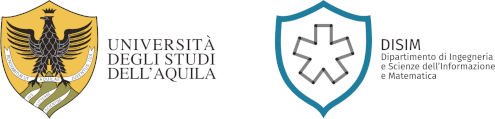## Home

I was born in Salerno in 1982 and I am currently Full Professor of Numerical Analysis at the Department of Information Engineering and Computer Science and Mathematics, University of L'Aquila. My research interests principally regard the numerical modeling for deterministic and stochastic evolutive problems, with special emphasis to geometric numerical integration. Specifically:

• structure-preserving numerical integration of deterministic and stochastic evolutionary problems (linear and non-linear oscillators; Hamiltonian problems; dissipative problems);
• linear and nonlinear stability of deterministic and stochastic numerical methods;
• adapted numerical methods for partial differential equations;
• numerical integration of deterministic and stochastic problems with memory (Volterra integral equations, fractional differential equations);
• numerical collocation techniques;
• exponential/trigonometrical numerical schemes for oscillatory problems;
• numerical schemes for stiff problems free from order reduction;
• numerical modeling of chemical oscillators;
• numerical modeling of fake news diffusion.

# Annunci

##### Message to international students of the Master’s Degrees in "Mathematical Engineering" and "Mathematical Modelling"

Dear international students of the Master's Degree in "Mathematical Enginnering" and "Mathematical Modelling" first of all, welcome in our programs! First years of these two ...

##### Inizio lezioni primo anno LM in Ingegneria Matematica e primo anno LM in Mathematical Modelling

Il prossimo 19 settembre 2022 inizieranno le lezioni del primo anno LM in Ingegneria Matematica (I4W) e primo anno LM in Mathematical Modelling (I4Y) per l'A.A. 2022-23. Per la ...

##### Ph.D. defence - Stefano Di Giovacchino (Ph.D. program in Mathematics and Models - XXXIV cycle)

Dear All I am pleased to announce that Stefano Di Giovacchino (Ph.D. program in Mathematics and Models - XXXIV cycle) will defend his Ph.D. thesis on June 23, 2022 at ...

Utilizziamo i cookie per offrirti il ​​nostro servizio. Continuando a utilizzare questo sito acconsenti al nostro utilizzo dei cookie come descritto nella nostra policy.